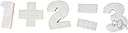# mathematical statement

Also found in: Thesaurus, Medical, Legal, Encyclopedia, Wikipedia.
ThesaurusAntonymsRelated WordsSynonymsLegend:
 Noun 1mathematical statement - a statement of a mathematical relationmath, mathematics, maths - a science (or group of related sciences) dealing with the logic of quantity and shape and arrangementequation - a mathematical statement that two expressions are equalstatement - a message that is stated or declared; a communication (oral or written) setting forth particulars or facts etc; "according to his statement he was in London on that day"formula, expression - a group of symbols that make a mathematical statementmathematical notation - a notation used by mathematicians
Based on WordNet 3.0, Farlex clipart collection. © 2003-2012 Princeton University, Farlex Inc.
References in periodicals archive ?
The mathematical statement of such problems leads to an inverse problem for the heat equation, where it is required to find not only a solution of the problem, but also its right-hand side that depends only on a spatial variable.
For example, Sorin Bangu (2008) argues that the explanandum of Baker's cicada example is a mathematical statement, so the example is not a mathematical explanation of a concrete phenomenon but a mathematical explanation of a mathematical phenomenon.
However, throughout the book she reminds students of the importance of the logical structure of a mathematical statement as a framework for finding a proof of that statement.
In general, a mathematical statement may contain any number of logical connectives--and, or, if-then--as well as the two quantifiers--for all, there exists.
a mathematical statement can be proved or disproved.
For this particular kind of number talk the teacher chooses a mathematical statement and poses the question: "Is this true or false?" The teacher then conducts the discussion using the actions described above.
The mathematical statement of the phase unwrapping problem can be expressed by
Even if not all true statements can be proved, is there always a way to decide whether a given mathematical statement is provable or not?
Is it a speculative 'subject' of the mathematical statement, which has no place in the factial but nevertheless can speak in it, that remains?
In his essay "Fictionalism, Theft, and the Story of Mathematics," Mark Balaguer proposes, among other arguments, that rather than describe a mathematical statement as "true," it could be described as "true within the story of mathematics."
The role of the symbolic geometry technology in both examples lay in allowing the user first to explore the problem visually, then in assisting in the conversion of this visual "feel" into a sharp mathematical statement, expressed symbolically.
After each mathematical statement, the author immediately cites a real-world example to model his mathematical imagery for the reader--a refreshing visualization device for individuals unable to grasp the math quickly.

Site: Follow: Share:
Open / Close